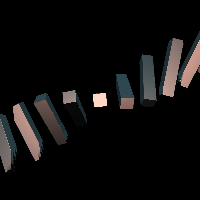# rangeTransform

filter
rangeTransform
RayTK
v0.36

Applies a transform based on a range of settings, mapped with either the iteration value or a field input.

This operator defines two different sets of transform settings. It then uses some index value to decide where in that range it will use. A simple use case for this would be iteration with 3 items, where the first item moves to one position and the last item moves to another position, and the one in the middle moves to half way between those two.

The index is based either on the first (x) part of the iteration value provided by a downstream op, or if the second input is connected, that float value field is used to determine the index. The index is then scaled from the specified range down to 0..1. The `Extend` parameter controls how values outside that range are handled.

## Parameters

• `Enable`:
• `Index Range`: The range of index values that are expected. The first value will use `Translate1` and the second will use `Translate2`.
• `Extend Mode`: How to handle index values outside of `Indexrange`.
• `Linear`: Linearly scale values, which is like making a graph with a line between the two settings, and then just extending that line in the same direction as it goes past 1 (and before 0).
• `Clamp`: Clamp the index values to the range.
• `Loop`: Loop the values over the range.
• `Enable Translate`: Wheteher to use translation.
• `Translate 1`: Translate setting for the beginning of the range.
• `Translate 2`: Translate setting for the end of the range.
• `Enable Rotate`: Whether to use rotation.
• `Rotate 1`: Rotate setting for the beginning of the range.
• `Rotate 2`: Rotate setting for the end of the range.
• `Use Pivot`:
• `Pivot 1`:
• `Pivot 2`:

## Inputs

• `definition_in`: (optional)
• Coordinate Types: `vec2` `vec3`
• Context Types: `Context` `MaterialContext` `CameraContext` `LightContext` `RayContext` `ParticleContext`
• Return Types: `float` `vec4` `Sdf` `Ray` `Light` `Particle`
• `Index Field`: (optional) Optional float value field to use instead of the iteration value. It is passed the current coordinates.
• Coordinate Types: `vec2` `vec3`
• Context Types: `Context` `MaterialContext` `CameraContext` `LightContext` `RayContext` `ParticleContext`
• Return Types: `float`
• `Easing`: (optional)
• Coordinate Types: `float`
• Context Types: `Context` `MaterialContext` `CameraContext` `LightContext` `RayContext` `ParticleContext`
• Return Types: `float`